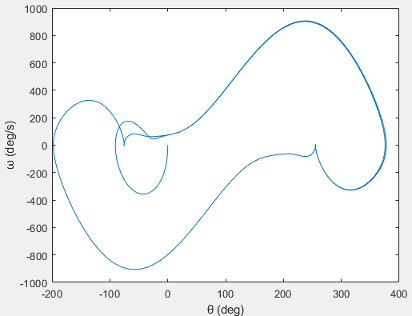## Analyze a Simple Pendulum

In this example, you explore the forces and torques that you can add to a model. Then you use blocks that have motion-sensing capabilities to analyze the dynamic response of the model. The end result is a set of time-domain and phase plots, one for each combination of forces and torques. You create these plots by using MATLAB® commands with Simscape™ Multibody™ motion outputs as arguments.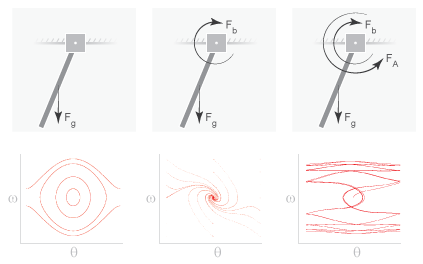First, create the simple pendulum model in Model a Simple Pendulum. By adding forces and torques to this model, you incrementally change the pendulum from undamped and free to damped and driven. The forces and torques that you apply include:

• Gravitational force (Fg) — Global force that acts on every body in direct proportion to its mass,which you specify in terms of the acceleration vector g. You specify this vector using the Mechanism Configuration block.

• Joint damping (Fb) — Internal torque between the pendulum and the joint fixture which you parameterize in terms of a linear damping coefficient. You specify this parameter using the Revolute Joint block that connects the pendulum to the joint fixture.

• Actuation torque (FA) — Driving torque between the pendulum and the joint fixture, which you specify directly as a Simscape physical signal using the Revolute Joint block that connects the pendulum to the joint fixture.

### Output Sensing Information for the Pendulum Motion

First, update the `simple_pendulum` model to output position and velocity data, then output this data to the MATLAB base workspace.

1. Open the `simple_pendulum` model that you created in tutorial Model a Simple Pendulum.

2. In the Z Revolute Primitive (Rz) > Sensing section of the Revolute Joint block dialog box, select the following parameters:

• Position

• Velocity

The block exposes two additional physical signal ports, q and w, that output the angular position and velocity of the pendulum with respect to the world frame.

3. Add these blocks to the model. You use the blocks to output the joint position and velocity to the MATLAB base workspace.

LibraryBlockQuantity
Simscape > UtilitiesPS-Simulink Converter2
Simulink > SinksTo Workspace2

4. Change the parameters in the To Workspace block dialog boxes to `q` and `w`. These variables make it easy to identify the joint variables that the To Workspace blocks output during simulation—position, through the Revolute Joint block port q, and velocity, through the Revolute Joint block port w. To remove the keyword, `out`, from the variable names, on the tab of the Simulink® Toolstrip, click Model Settings, then select Data Import/Export and clear the Single simulation output parameter.

5. Connect the blocks as shown in the figure. Ensure that the To Workspace block with the variable name `q` connects, through the PS-Simulink Converter block, to the Revolute Joint block port q, and that the To Workspace block with variable name `w` connects to the Revolute Joint block port w.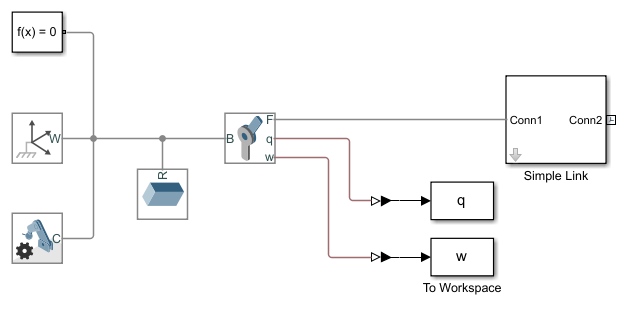6. Change the value of the Vector format parameter to `1-D array` for both PS-Simulink Converter blocks.

7. Save the model under a different name, such as `simple_pendulum_analysis`.

### Analyze Undamped Pendulum

1. Run the simulation. Mechanics Explorer opens with a 3-D animation of the simple pendulum model.

2. Plot the joint position and velocity with respect to time by entering the following code at the MATLAB command prompt:

```figure; hold on; plot(q); plot(w); ```
The figure shows the resulting plot.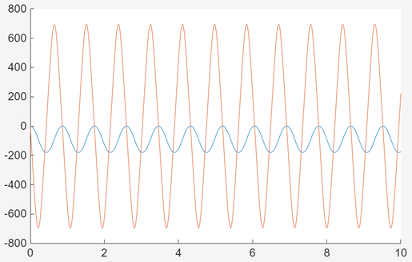3. Plot the joint angular velocity with respect to the angular position by entering this code at the MATLAB command prompt.

```figure; plot(q.data, w.data); ```
The result is the phase plot of the joint that corresponds to a starting position of zero degrees with respect to the horizontal plane.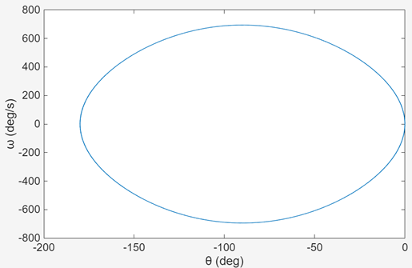Try simulating the model using different starting angles. In the Z Revolute Primitive (Rz) > State Targets section, under Specify Position Target, specify the Value parameter of the Revolute Joint block dialog box. This figure shows a compound phase plot for starting angles of -80, -40, 0, 40, and 80 degrees.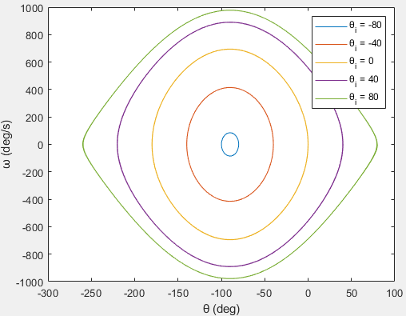### Analyze Damped Pendulum

1. In the Revolute Joint block dialog box, set Z Revolute Primitive (Rz) > Internal Mechanics > Damping Coefficient to `8e-5` (N*m)/(deg/s). The damping coefficient causes energy dissipation during motion which results in a gradual decay of the pendulum oscillation amplitude.

2. Ensure that Z Revolute Primitive (Rz) > State Targets > Specify Position Target > Value is set to `0` deg.

3. Run the simulation.

4. Plot the joint position and velocity with respect to time. To do this, enter this code at the MATLAB command prompt.

```figure; hold on; plot(q); plot(w);```
The figure shows the resulting plot. Note that the pendulum oscillations decay with time due to damping. At larger damping values, the pendulum becomes overdamped and the oscillations disappear.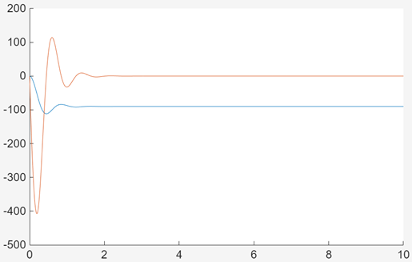5. Plot the joint phase plot. To do this, enter this code at the MATLAB command prompt.

```figure; plot(q.data, w.data);```
The figure shows the resulting plot.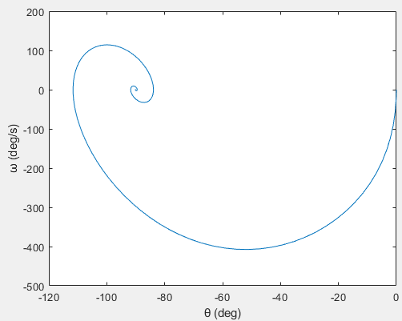Try simulating the model using different starting angles. In the Z Revolute Primitive (Rz) > State Targets section, under Specify Position Target, specify Value parameter of the Revolute Joint block dialog box. The figure shows a compound phase plot for starting angles of `-240`, `-180`, `-120`, `-60`, `0`, and `60` degrees.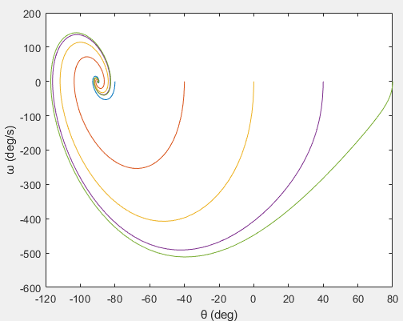### Analyze Damped and Driven Pendulum

1. In the Revolute Joint block dialog box, in the Actuation section, set the Torque parameter to ```Provided by Input```. The block exposes a physical signal input port, t, that you can use to specify the joint actuation torque.

2. Add these blocks to the model.

LibraryBlock
Simscape > UtilitiesSimulink-PS Converter
Simulink > SourcesSine Wave

The Sine Wave block provides a periodic torque input as a Simulink signal. The Simulink-PS Converter block converts the Simulink signal to a physical signal compatible with Simscape Multibody blocks.

3. Connect the blocks as shown in the figure.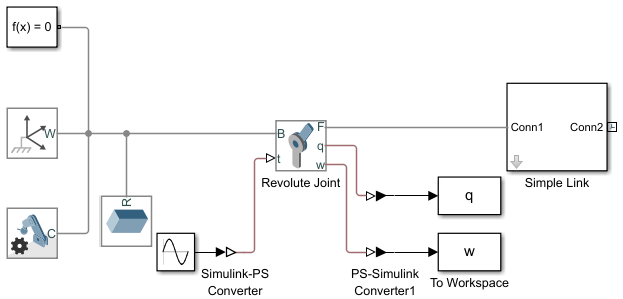4. In the Sine Wave block dialog box, set the Amplitude parameter to `0.06`. This amplitude corresponds to an actuation torque that oscillates between `-0.06` N and `0.06` N.

5. In the Revolute Joint block dialog box, in the Z Revolute Primitive (Rz) > State Targets section, under Specify Position Target,set Value parameter to `0` deg.

6. Run the simulation.

7. Plot the joint position and velocity with respect to time. To do this, enter this code at the MATLAB command prompt.

```figure; hold on; plot(q); plot(w);```
The figure shows the resulting plot.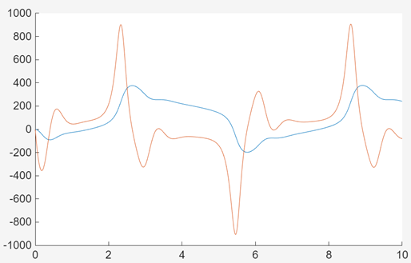8. Plot the joint phase plot. To do this, enter this code at the MATLAB command prompt:

```figure; plot(q.data, w.data);```
The figure shows the resulting plot.